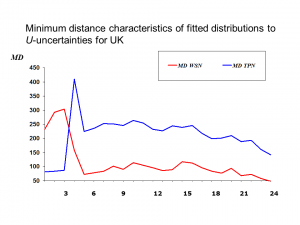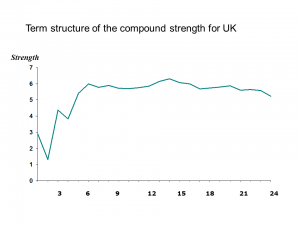# Probabilistic Approach to Assessing Macroeconomic Uncertainties

PRAMU

## Inflation uncertainty for the United Kingdom

Posted on: June 3rd, 2015 by pramu No Comments

# Inflation uncertainty for the United Kingdom

1. Figure 1. Minimum distance characteristics of fitted TPN and WSN distributions to U-uncertaintiesWSN fits better than TPN except for very short forecast horizons.

2. Figure 2. Term structure of the compound strength of forecast-induced monetary policyThe compound strength is computed as: $Strength=|alpha|cdot D_m+|beta|cdot D_k$, where $D_a=int_{|a|}^infty t^2varphi(t)dt=1-Phi(|a|)+|a|varphi(a)$. In the policy-input symmetric case, where $m=-k$ (and this is the assumption applied for empirical estimation), the multipliers $D_a$ can be ignored.

3. Table 1. Forecast uncertainty measures

4. Figure 3. Ex-post and ex-ante inflation fan chartsEx-post uncertainty Ex-ante uncertainty